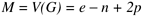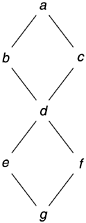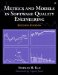# Cyclomatic Complexity

The measurement of cyclomatic complexity by McCabe (1976) was designed to indicate a program's testability and understandability (maintainability). It is the classical graph theory cyclomatic number, indicating the number of regions in a graph. As applied to software, it is the number of linearly independent paths that comprise the program. As such it can be used to indicate the effort required to test a program. To determine the paths, the program procedure is represented as a strongly connected graph with unique entry and exit points. The general formula to compute cyclomatic complexity is:where

 V ( G ) = Cyclomatic number of G e = Number of edges n = Number of nodes p = Number of unconnected parts of the graph

As an example, Figure 11.2 is a control graph of a simple program that might contain two IF statements. If we count the edges, nodes, and disconnected parts of the graph, we see that e = 8, n = 7, and p = 1, and that M = 8 “ 7 + 2 * 1 = 3.

Figure 11.2. Simple Control Graph ExampleNote that M is also equal to the number of binary decisions in a program plus 1. If all decisions are not binary, a three-way decision is counted as two binary decisions and an n -way case (select) statement is counted as n “ 1 binary decisions. The iteration test in a looping statement is counted as one binary decision. In the preceding simple example, since there are two binary decisions, M = 2 + 1 = 3.

The cyclomatic complexity metric is additive. The complexity of several graphs considered as a group is equal to the sum of the individual graphs' complexities. However, it ignores the complexity of sequential statements. Neither does the metric distinguish different kinds of control flow complexity such as loops versus IF-THEN-ELSE statements or cases versus nested IF-THEN-ELSE statements.

To have good testability and maintainability, McCabe recommends that no program module should exceed a cyclomatic complexity of 10. Because the complexity metric is based on decisions and branches, which is consistent with the logic pattern of design and programming, it appeals to software professionals. Since its inception, cyclomatic complexity has become an active area of research and practical applications. Many experts in software testing recommend use of the cyclomatic representation to ensure adequate test coverage; the use of McCabe's complexity measure has been gaining acceptance by practitioners .

Because of its appeal to programmers and researchers, many studies have been conducted to relate McCabe's complexity measure to defect rate, and moderate to strong correlations were observed . For instance, in a study of software metrics of a large SQL product that consisted of about 1300 modules, Troster (1992) found a relatively strong correlation between McCabe's cyclomatic complexity index and the number of test defects ( r = .48, n = 1303, p = .0001). Studies found that the complexity index also correlates strongly with program size ”lines of code. Will the correlation between complexity and defect remain significant after program size is controlled? In other words, is the correlation between complexity and defects a spurious one, because program size affects both complexity and defect level? Many studies have been done with regard to this question and the findings are not always consistent. There are cases where the correlation disappears after the effect of program size is controlled; in other cases the correlation weakens somewhat but remains significant, suggesting a genuine association between complexity and defect level. Our experience belongs to the latter kind.

Sometimes the disappearance of the correlation between complexity and defect level after accounting for program size may be due to a lack of investigational rigor. It is important that appropriate statistical techniques be used with regard to the nature of the data. For example, Troster observed that the LOC count also correlated with the number of test defects quite strongly ( r = 0.49, n = 1296, p = 0.001). To partial out the effect of program size, therefore, he calculated the correlation between McCabe's complexity index and testing defect rate (per KLOC). He found that the correlation totally disappeared with r = 0.002 ( n = 1296, p = 0.9415). Had Troster stopped there, he would have concluded that there is no genuine association between complexity and defect level. Troster realized, however, that he also needed to look at the rank-order correlation. Therefore, he also computed the Spearman's rank-order correlation coefficient and found a very respectable association between complexity and defect rate:

Spearman's correlation = 0.27

n = 1296 (number of modules)

p = 0.0001 (highly statistically significant)

These seemingly inconsistent findings, based on our experience and observation of the Troster study, is due to the nature of software data. As discussed previously, Pearson's correlation coefficient is very sensitive to extreme data points; it can also be distorted if there is a lot of noise in the data. Defect rate data (normalized to KLOC) tend to fluctuate widely and therefore it is difficult to have significant Pearson correlation coefficients. The rank-order correlation coefficient, which is less precise but more robust than the Pearson correlation coefficient, is more appropriate for such data.

As another example, Craddock (1987) reports the use of McCabe's complexity index at low-level design inspections and code inspection (I2). He correlated the number of inspection defects with both complexity and LOC. As shown in Table 11.2, Craddock found that complexity is a better indicator of defects than LOC at the two inspection phases.

Assuming that an organization can establish a significant correlation between complexity and defect level, then the McCabe index can be useful in several ways, including the following:

Table 11.2. Correlation Coefficients Between Inspection Defects and Complexity

Inspection Type

Number of Inspections

KLOC

r

Lines of Code

r

McCabe's Index

I0

46

129.9

0.10

I1

41

67.9

0.46

0.69

I2

30

35.3

0.56

0.68

• To help identify overly complex parts needing detailed inspections
• To help identify noncomplex parts likely to have a low defect rate and therefore candidates for development without detailed inspections
• To estimate programming and service effort, identify troublesome code, and estimate testing effort

Later in this chapter we describe an example of complexity study in more detail and illustrate how quality improvement can be made via the focus on complexity reduction.Metrics and Models in Software Quality Engineering (2nd Edition)
ISBN: 0201729156
EAN: 2147483647
Year: 2001
Pages: 176